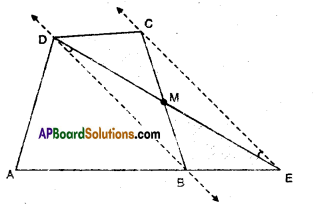# AP Board 9th Class Maths Solutions Chapter 11 Areas Ex 11.3

AP State Syllabus AP Board 9th Class Maths Solutions Chapter 11 Areas Ex 11.3 Textbook Questions and Answers.

## AP State Syllabus 9th Class Maths Solutions 11th Lesson Areas Exercise 11.3

Question 1.
In a triangle ABC, E is the midpoint of median AD. Show that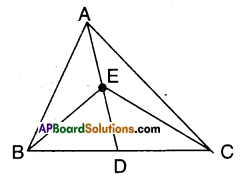i) ar ΔABE = ar ΔACE
Solution:
In ΔABC; AD is a median.
∴ ΔABD = ΔACD …………….. (1)
(∵ Median divides a triangle in two equal triangles)
Also in ΔABD; BE is a median.
∴ ΔABE = ΔBED = $$\frac{1}{2}$$ΔABD …………..(2)
Also in ΔACD; CE is a median.
∴ ΔACE = ΔCDE = $$\frac{1}{2}$$ΔACD …………….(3)
From (1), (2) and (3);
ΔABE = ΔACE

(OR)

ΔABD = ΔACD [∵ AD is median in ΔABC]
$$\frac{1}{2}$$ ΔABD = $$\frac{1}{2}$$ ΔACD
[Dividing both sides by 2]
ΔABE = ΔAEC
[∵ BE is median of ΔABD, CE is median of ΔACD]
Hence proved.

ii) arΔABE = $$\frac{1}{2}$$ ar(ΔABC)
Solution:
ΔABE = $$\frac{1}{2}$$ (ΔABD)
[From (i); BE is median of ΔABD]
ΔABE = $$\frac{1}{2}$$ [$$\frac{1}{2}$$ ΔABC]
[∵ AD is median of ΔABC]
= $$\frac{1}{4}$$ ΔABC
Hence,proved.Question 2.
Show that the diagonals of a paral¬lelogram divide it into four triangles of equal area.
Solution:
□ABCD is a parallelogram.
The diagonals AC and BD bisect each other at ‘O’.
ΔABC and □ABCD lie on the same base AB and between the same parallels AB//CD.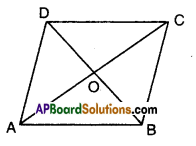∴ ΔABC = $$\frac{1}{4}$$ □ABCD
Now in ΔABC; BO is a median
[∵ O is the midpoint of both diagonals AC and BD]
∴ ΔAOB = ΔBOC ………….(1)
[ ∵ Median divides a triangle into two triangles of equal area]
Similarly ∵ABD and □ABCD lie on the same base AB and between the same parallels AB//CD.]
∴ ΔABD = $$\frac{1}{2}$$□ ABCD
Also ΔAOB = ΔAOD …………..(2)
[ ∵ AO is the median of AABD]
From (1) & (2)
ΔAOB = ΔBOC = ΔAOD
Similarly we can prove that
ΔAOD = ΔCOD [ ∵ OD is the median of ΔACD]
∴ ΔAOB = ΔBOC = ΔCOD = ΔAOD
Hence proved.Question 3.
In the figure, ΔABC and ΔABD are two triangles on the same base AB. If line segment CD is bisected by $$\overline{\mathbf{A B}}$$ at O, show that ar (ΔABC) = ar (ΔABD).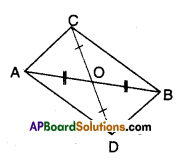Solution:
From the figure, in ΔAOC; ΔBOD
OA = OB [ ∵ given]
∠AOC = ∠BOD [Vertically opp. angles]
∴ ΔAOC ≅ ΔBOD (SAS congruence)
Thus AC = BD (CPCT)
∠OAC = ∠OBD (CPCT) .
But these are alternate interior angles for the lines AC, BD.
∴ AC // BD
As AC = BD and AC // BD;
□ABCD is a parallelogram.
AB is a diagonal of oABCD
⇒ ΔABC ≅ ΔABD
(∵ diagonal divides a parallelogram into two congruent triangles)
∴ ar(ΔABC) = ar (ΔABD)Question 4.
In the figure ΔABC; D, E and F are the midpoints of sides BC, CA and AB respectively. Show that
i)BDEF is a parallelogram
ii) ar (ΔDEF) = $$\frac { 1 }{ 4 }$$ ar(ΔABC)
iii) ar (BDEF) = $$\frac { 1 }{ 2 }$$ ar(ΔABC)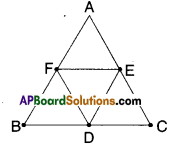Solution:
i) In ΔABC; D, E and F are the mid¬points of the sides.
∴ EF//BC FD//AC ED//AB
EF = $$\frac { 1 }{ 2 }$$BC FD = $$\frac { 1 }{ 2 }$$AC ED = $$\frac { 1 }{ 2 }$$AB
[ ∵ line joining the mid points of any two sides of a triangle is parallel to third side and equal to half of it]
∴ In □BDEF
BD = EF [ ∵ D is mid point of BC and $$\frac { 1 }{ 2 }$$ BC = EF]
DE = BF
∴ □BDEF is a parallelogram.

ii) □BDEF is a parallelogram (from (i))
Thus ΔBDF = ΔDEF
Similarly □CDFE; □AEDF are also parallelograms.
∴ ΔDEF = ΔCDE =ΔAEF
∴ ΔABC = ΔAEF+ ΔBDF + ΔCDF + ΔDEF
= 4ΔDEF
⇒ ΔDEF = $$\frac { 1 }{ 4 }$$ΔABC

iii) □BDEF = 2 ΔDEF …………..(1)
(from (ii))
ΔABC = 4 ΔDEF (2)
(from (ii))
From (1) & (2);
ΔABC = 2 (2ΔDEF) = 2 □BDEF
Hence proved.Question 5.
In the figure D, E are points on the sides AB and AC respectively of ΔABC such that ar (ΔDBC) = ar (ΔEBC). Prove that DE // BC.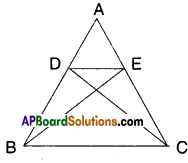Solution:
ΔDBC = ΔEBC
The two triangles are on the same base BC and between the same pair of lines BC and DE.
As they are equal in area.
∴ BC // DE.

Question 6.
In the figure, XY is a line parallel to BC is drawn through A. If BE // CA and CF // BA are drawn to meet XY at E and F respectively. Show that ar (ΔABE) = ar (ΔACF).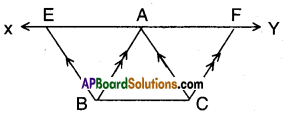Solution:
Given that XY//BC; BE//CA; CF//BA
In quad ABCF; AB//CF and BC//AF
Hence □ABCF is a parallelogram.
Also in □ACBE ; BC//AE and AC//BE
Hence □ACBE is a parallelogram.
Now in □ABCF and □ACBE
ΔABC = ΔACF …………..(1);
ΔABC = ΔABE …………..(2)
[∵ Diagonal divides a parallelogram into two congruent triangles]
∴ ΔACF = ΔABE [from (1) & (2)]
Hence proved.Question 7.
In the figure, diagonals AC and BD of a trapezium ABCD with AB//DC inter¬sect each other at ‘O’. Prove that ar (ΔAOD) = ar (ΔBOC)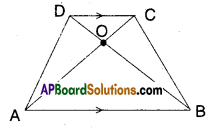Solution:
Given that AB // CD
Now ΔADC and ΔBCD are on the same base and between the same parallels AB // CD.
⇒ ΔADC – ΔCOD = ΔBCD – ΔCOD
⇒ ΔAOD = ΔBOC [from the figure]

Question 8.
In the figure ABCDE is a pentagon. A line through B parallel to AC meets DC produced at F. Show that
(i) ar (ΔACB) = ar (ΔACF)
(ii) ar (AEDF) = ar (ABCDE)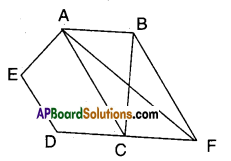Solution:
ABCDE is a pentagon.
AC//BF
i) ΔACB and ΔACF are on the same base AC and between the same parallels AC//BF.
∴ ΔACB = ΔACF

ii) □AEDF = □AEDC + ΔACF
= □AEDC + ΔABC
[ ∵ ΔACF = ΔACB]
= area (ABCDE)
Hence proved.Question 9.
In the figure, if ar (ΔRAS) = ar (ΔRBS) and ar (ΔQRB) = ar (ΔPAS) then show that both the quadrilaterals PQSR and RSBA are trapeziums.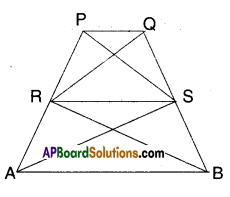ΔRAS = ΔRBS ………….. (1)
Both the triangles are on the same base RS and between the same pair of lines RS and AB.
As their areas are equal RS must be parallel to AB.
⇒ RS//AB
∴ □ABRS is a quadrilateral in which AB//RS.
∴ □ABRS (or) □RSBA is a trapezium.
Now AQRB = APAS (given)
⇒ ΔQRB – ΔRBS = ΔPAS – ΔRAS
[from (1) ΔRBS = ΔRAS]
⇒ ΔQRS = ΔPRS
These two triangles are on the same base RS and between the same pair of lines RS and PQ.
As these two triangles have same area RS must be parallel to PQ.
⇒ RS // PQ
Hence □PQRS is a trapezium.Question 10.
A villager Ramayya has a plot of land in the shape of a quadrilateral. The grampanchayat of the village decided to take over some portion of his plot from one of the comers to construct a school. Ramayya agrees to the above proposal with the condition that he should be given equal amount of land in exchange of his land adjoining his plot so as to form a triangular plot. Explain how this proposal will implemented. (Draw a rough sketch of the plot.)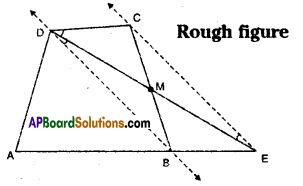Solution:
Let □ABCD is the plot of Ramayya.
School be constructed in the region ΔMCD where M is a point on BC such that □ABCD ≅ ΔADE
Draw the diagonal BD.
Draw a line parallel to BD through C which meets AB produced at E.
Join D, E